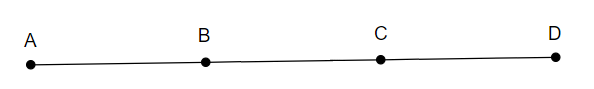# If $\mathrm{B}$ is the mid point of $\overline{\mathrm{AC}}$ and $\mathrm{C}$ is the mid point of $\overline{\mathrm{BD}}$, where $\mathrm{A}, \mathrm{B}, \mathrm{C}, \mathrm{D}$ lie on a straight line, say why $\mathrm{AB}=\mathrm{CD}$?

Given:

$\mathrm{B}$ is the mid point of $\overline{\mathrm{AC}}$ and $\mathrm{C}$ is the mid point of $\overline{\mathrm{BD}}$, where $\mathrm{A}, \mathrm{B}, \mathrm{C}, \mathrm{D}$ lie on a straight line.

To do:

We have to say why $\mathrm{AB}=\mathrm{CD}$.

Solution:From the figure,

B is the midpoint of $AC$.

This implies,

$AB = BC$..........(i)

C is the midpoint of BD.

This implies,

$BC = CD$............(ii)

From (i) and (ii), we get,

$AB = CD$

Hence proved.